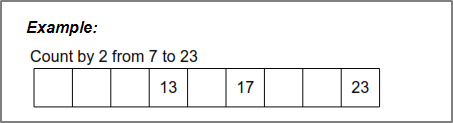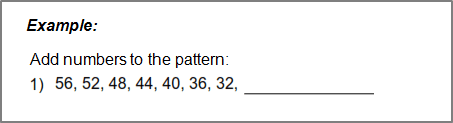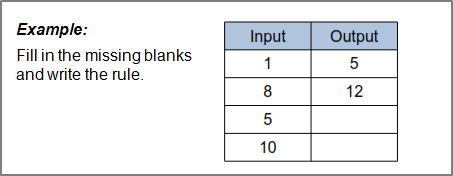# Grade 1 Number Pattern Worksheets

## Number and counting patterns worksheets

Our grade 1 number patterns numbers worksheets provide exercises in analyzing and extending number patterns.  All patterns are based on simple addition or subtraction and all are 'single-step' patterns.  Numbers up to 100 are used.

## Counting patterns

The student is given a simple pattern and rule and must fill in the missing numbers.We have 6 counting pattern worksheets  categorized as ascending / descending, counts up to 5 or 9 and numbers up to 50 or 100.

## Number Patterns: extending the pattern

The student is given a pattern of 7 numbers and is asked to extend the pattern with additional numbers.  The student is not explicitly told the "rule" and has to figure out the relationship between the numbers himself.Our extending number patterns worksheets are both ascending and descending and have numbers up to 100.

## Number patterns: identifying the pattern and filling in the missing numbers

The student is given a pattern of numbers with over half the numbers missing.  The student must figure out the relationship between consecutive numbers (which will be simple addition or subtraction) and fill in the missing blanks.  Numbers up to 100 are used.

These identifying number pattern worksheets have 7-10 numbers per pattern.

## Number patterns: input / output charts

The student is given a chart showing a pattern of "input" and "output" numbers and must determine the relationship between them (which will be simple addition or subtraction) so that he/she can fill in the missing numbers.  Numbers up to 100 are used.These 6 input - output charts are based in simple addition / subtraction (or skip counting).

## More numbers worksheets

Explore all of our learning numbers worksheets (recognizing and printing numbers), counting worksheets (counting objects, skip counting, counting backwards) and comparing numbers worksheets ("more than", "less than", ordering numbers).

## What is K5?

K5 Learning offers reading and math worksheets, workbooks and an online reading and math program for kids in kindergarten to grade 5.  We help your children build good study habits and excel in school.